Hello Guest it is January 20, 2022, 03:40:59 AM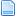Author Topic: Angle calculations for G-Code?  (Read 12326 times)

0 Members and 1 Guest are viewing this topic.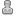Rimmel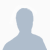•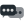168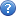Angle calculations for G-Code?
« on: January 25, 2014, 12:12:24 PM »
I have been searching for a while on how to calculate and angle in g-code.

e.g.

Stock - 50 diameter

I want to cut a a 45 degree angleThe mach3 wizards with cut a taper but the g-code overshoots the end. So if I put a x as 10 and z as 0 the the code will go to -9.5 (example), this is OK if it's an end angle but not if it's like the picture.

Questions:
I've seen a couple of wizards that ask for angle and then start z and end z. Isn't the z end redundant? e.g if i wanted a angle from x=0 z=0 @45 degrees to a stock diameter of 30mm then put in a z end of 500mm the angle using the z end would not be 45 degrees. I would have thought it would be either an angle or point to point and you get what angle comes out.

Anyway I would like the formulas for working this out myself - arc formulas would be nice as well.

thanks
« Last Edit: January 25, 2014, 12:22:36 PM by Rimmel »RICH••7,422Re: Angle calculations for G-Code?
« Reply #1 on: January 25, 2014, 01:47:37 PM »
Rimmel,

Do an internet search "Right Triangle Formulas"
Similar for arc's

You'll definately find something that suites your math understanding.

RICHGraham Waterworth•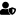•2,437
• Yorkshire Dales, EnglandRe: Angle calculations for G-Code?
« Reply #2 on: January 27, 2014, 07:39:11 AM »
If you are working in diameter mode then the code is simply move to start diameter and Z start e.g. X30. Z2., feed in 10mm G01 Z-10. F.1 then form a 10*45 deg chamfer X50. Z-20. this may be a big chamfer to cut in 1 go but that's the code to do it. so for 45 degree chamfer move twice the x for the z e.g. a z move of 2 will need and x move of 4.

G00 X30. Z2. G97 S1000 M3
G01 Z-10. F.1
X50. Z-20.
X52.
G00 Z2.
M30

For a 30 degree chamfer the x and z are the same e.g. x2. z-2. will create a 30 degree chamfer.
Without engineers the world stops